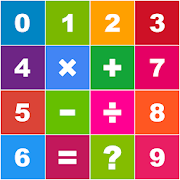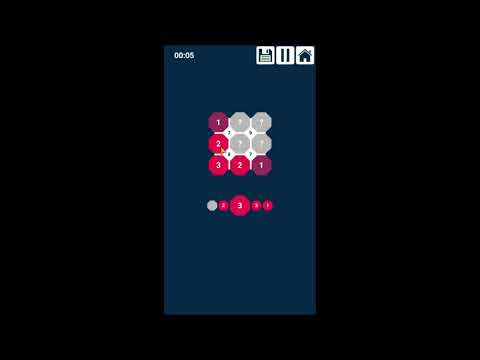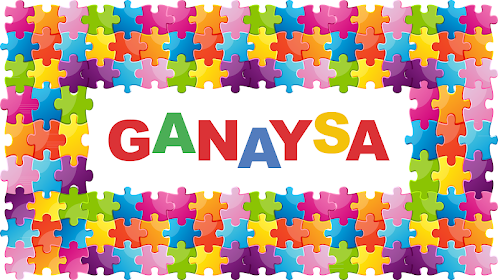# Arithmetic Math Games CollectionEveryone
·
Offers in-app purchasesThis is a collection of 11 arithmetic games to test your mental math skills. Best math practice for the whole family kids and adults.

List of games:
★ Arithmetic Addition: Connect 3 or more numbers so that their sum is equal to one of the target numbers.

★ Arithmetic Multiplication: Connect 3 or more numbers so that the result of their multiplication is equal to one of the target numbers.

★ Arithmetic Division: Connect 3 or more numbers so that the result of their division is equal to one of the target numbers.

★ Arithmetic Subtraction: Connect 3 or more numbers so that the result of their subtraction is equal to one of the target numbers.

★ 1 + 2 = 3: It’s a simple math addition and subtraction game where its equations consist of the numbers 1, 2 and 3 and its result is also 1, 2 or 3. Tap the correct answer.

★ 1 + 2 = 3 Duel: It’s a simple math addition and subtraction game where 2 persons are competing on the same device. The score will be counted for the person who touches the correct answer first.

★ Arithmetic Challenge: It’s a math addition, subtraction, division and multiplication game where its equations consist of the numbers between 1 and 99 and its result is also a number between 1 and 99. Tap the correct answer.

★ Arithmetic Duel: It’s a math addition, subtraction, division and multiplication game where its equations consist of the numbers between 1 and 99 and its result is also a number between 1 and 99. 2 persons are competing on the same device. The score will be counted for the person who touches the correct answer first.

★ Crossword Sudoku Addition: Fill in the empty circles (octagons) so that the number between the four adjacent circles is the result of their sum. But be careful a number can't be used more than once in any column or row like in Sudoku.

★ Crossword Sudoku Multiplication: Fill in the empty circles (octagons) so that the number between the four adjacent circles is the result of their multiplication. But be careful a number can't be used more than once in any column or row like in Sudoku.

★ Dominoes Make 20: Drag and place dominoes on the grid so that the sum of dots of the non-empty rows or columns = 20

The game supports 14 languages: English – Arabic – French – Spanish – German – Japanese – Chinese – Urdu – Hindi – Italian – Portuguese – Turkish – Russian – Korean. The translations are done using Google Translate for all languages except English and Arabic. If you’d like to participate in translating the game content into your native language, please send an email to: playstore.ganaysa@gmail.com

Play, enjoy and have fun !

---------------
Collapse

Review Policy

## What's New

Some fixes
Collapse

Updated
December 8, 2019
Size
Varies with device
Installs
50+
Current Version
Varies with device
Requires Android
2.3 and up
Content Rating
Everyone
In-app Products
\$2.99 per item
Permissions
Offered By
Ganaysa
Developer